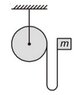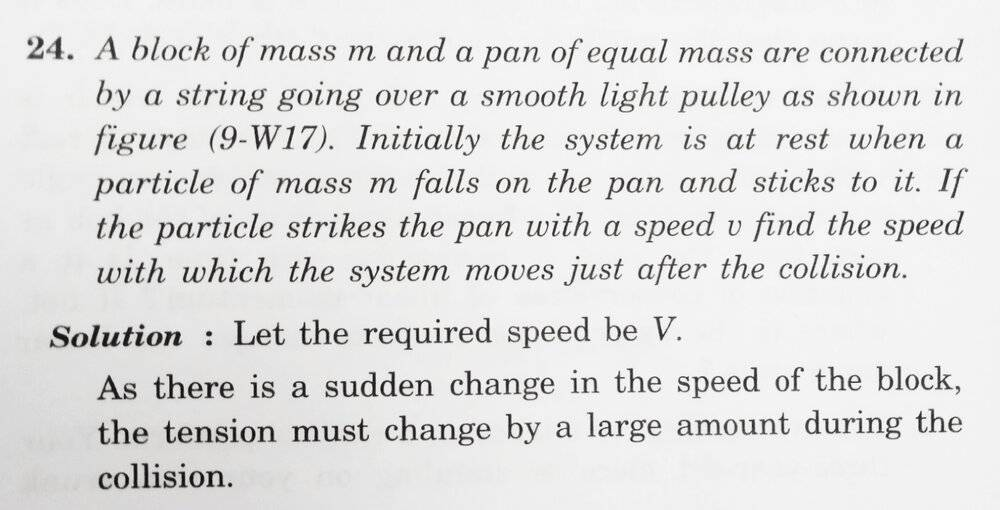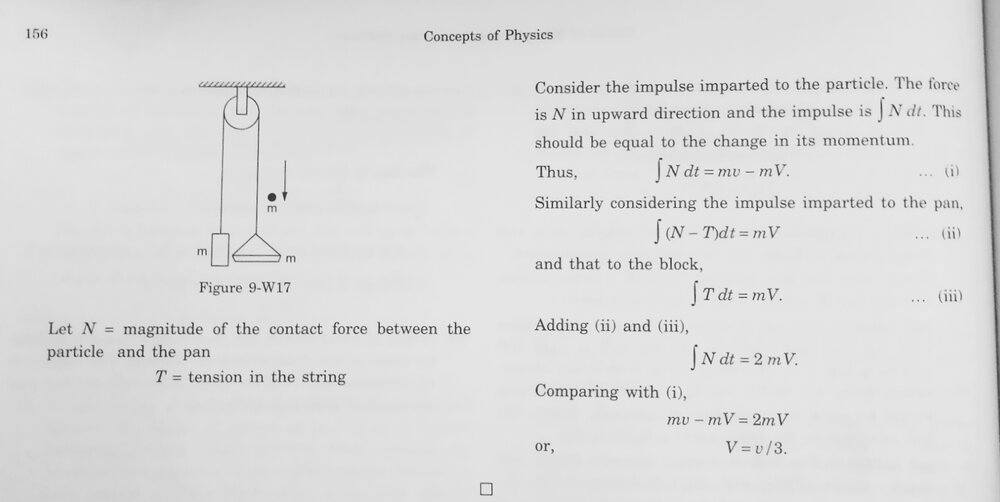# To find the angular momentum of a disc

PSN03
Homework Statement:
A block of mass m is attached to one end of a light string which is wrapped on a disc of mass 2m and radius R. The total length of the slack portion of the string is l. The block is released from rest. The angular velocity of the disc just after the string becomes taut is:
Relevant Equations:
Conservation of angular momentum
Initial angular momentum=final angular momentum
I was first wondering wether we can solve this question by applying conservation or energy or not but after googling it I found that we can't apply conservation of energy since there will be some energy lost in this case. I don't know how this energy is getting lost.
My second doubt was if we apply conservation of angular momentum about centre of the disc then won't there be a torque due to the string? So it won't be valid to use conservation of angular momentum.
Can anyone please clarify these doubts.

#### Attachments

•WCVv6.jpg
3.7 KB · Views: 200

Gold Member
In the figure attached is the distance between the center of pulley and the ( center of mass of ) block is R or more ? That may affect the answer.

Last edited:
Frigus
I was first wondering wether we can solve this question by applying conservation or energy or not but after googling it I found that we can't apply conservation of energy since there will be some energy lost in this case. I don't know how this energy is getting lost.
It depends upon question,
if it given that no energy is lost during the process then you can apply Law of conservation of energy but in case it is not going given then we assume it to be ideal.
My second doubt was if we apply conservation of angular momentum about centre of the disc then won't there be a torque due to the string? So it won't be valid to use conservation of angular momentum.
Yes you are right.
I think more relevant equation should be
##ω_f## = ##ω_i## + ##\frac {τ}{I}## ##t##.
Try solving it using this equation.

•PSN03
PSN03
In the figure attached is the distance between the center of pulley and the ( center of mass of ) block is R or more ?
How does it even matter. The potential energy is what we are concerned about. I don't think it's a useful information.

PSN03
It depends upon question,
if it given that no energy is lost during the process then you can apply Law of conservation of energy but in case it is not going given then we assume it to be ideal.

Yes you are right.
I think more relevant equation should be
##ω_f## = ##ω_i## + ##\frac {τ}{I}## ##t##.
Try solving it using this equation.
According to the solution I have we can't apply conservation of energy. If I do so then I will get a different answer. A similar question was asked on another website and the reason given by a user was
'The thing is that you can't use conservation of energy law in this case. At the moment when the string became taut some kind of inelastic impact would happen and some portion of energy would lost. Imagine, that the string is made of elastic rubber so that no energy would be lost. But in this case there will be some oscillations. In case the string is rigid these oscillations will fade out quickly, but some energy will be lost in the process.'
Though I didn't get much of this explanation as to where will the ineleastic impact happen and between which two bodies.

PSN03
It depends upon question,
if it given that no energy is lost during the process then you can apply Law of conservation of energy but in case it is not going given then we assume it to be ideal.

Yes you are right.
I think more relevant equation should be
##ω_f## = ##ω_i## + ##\frac {τ}{I}## ##t##.
Try solving it using this equation.
Yeah but the answer will be different if I use this. According to them there is no torque. I guess they are just making some assumptions to get their desired answer.

PSN03
It depends upon question,
if it given that no energy is lost during the process then you can apply Law of conservation of energy but in case it is not going given then we assume it to be ideal.

Yes you are right.
I think more relevant equation should be
##ω_f## = ##ω_i## + ##\frac {τ}{I}## ##t##.
Try solving it using this equation.
Initial angular velocity=0
w=0+(mg*R/2m*R²/2)*t
t=distance/velocity
=l/√2gl
=√l/2g

Now hen I plug these values I will get final angular momentum which is different from the actual answer

Frigus
Initial angular velocity=0
w=0+(mg*R/2m*R²/2)*t
t=distance/velocity
=l/√2gl
=√l/2g

Now hen I plug these values I will get final angular momentum which is different from the actual answer
Torque was acting only when string was taut.

PSN03
Torque was acting only when string was taut.
Yes ...totally agree..before that it was just a freely falling body

Frigus
Yes ...totally agree..before that it was just a freely falling body
So when you should take t=0?

•PSN03
PSN03
So when you should take t=0?
Ohh sorry...t=0 should be the time when the block has reached the bottomost point and the string is about to be taut😅

Frigus
Ohh sorry...t=0 should be the time when the block has reached the bottomost point and the string is about to be taut😅

Homework Helper
Gold Member
2022 Award
At the moment when the string became taut some kind of inelastic impact would happen
It's a bit more complicated than that. We are not told the string is inelastic. (We are not told it is inextensible either, but that's another matter.)
But suppose it is fairly elastic, though maybe with such a high constant that it is almost inextensible. Some of the energy will go into stretching the string, but thence into oscillations. So although some energy is absorbed by the string's inelasticity, some goes into oscillations which do not contribute to the overall subsequent motion.

•PSN03
PSN03
I don't know how but it's coming zero as all variables become zero
Initial angular momentum is zero
Time is also zero
w=0+k*0
=0
Where k is angular acceleration

PSN03
It's a bit more complicated than that. We are not told the string is inelastic. (We are not told it is inextensible either, but that's another matter.)
But suppose it is fairly elastic, though maybe with such a high constant that it is almost inextensible. Some of the energy will go into stretching the string, but thence into oscillations. So although the energy some is absorbed by the string's inelasticity, some goes into oscillations which do not contribute to the overall subsequent motion.
Ohk...this clears my first doubt...thanks a lot.
But what about the 2nd one, won't there be torque acting ?

Homework Helper
Gold Member
2022 Award
Ohk...this clears my first doubt...thanks a lot.
But what about the 2nd one, won't there be torque acting ?
While the block is falling there is no tension in the string so no torque.
What is the velocity just before it becomes taut? What angular momentum does that give the block about the disc's centre?

•PSN03
PSN03
While the block is falling there is no tension in the string so no torque.
What is the velocity just before it becomes taut? What angular momentum does that give the block about the disc's centre?
I clearly understood what you are trying to say here but my doubt is when the string becomes taut won't there be any kind of force acting and hence producing torque about centre?

Frigus
I don't know how but it's coming zero as all variables become zero
Initial angular momentum is zero
Time is also zero
w=0+k*0
=0
Where k is angular acceleration
It is the answer of this problem,
Doesn't it matches with answer key?

PSN03
It is the answer of this problem,
Doesn't it matches with answer key?
w=√gl/2R

Homework Helper
Gold Member
2022 Award
I clearly understood what you are trying to say here but my doubt is when the string becomes taut won't there be any kind of force acting and hence producing torque about centre?
You want the motion immediately after it becomes taut. There is no time for any continuing torques to affect it.

•PSN03
PSN03
You want the motion immediately after it becomes taut. There is no time for any continuing torques to affect it.
So you mean to say that just before the tension in the string starts to act and produce some torque we are finding the angular velocity...right?😅

Homework Helper
Gold Member
2022 Award
So you mean to say that just before the tension in the string starts to act and produce some torque we are finding the angular velocity...right?😅
You need to find the angular momentum of the system about the disc's centre just before tautness (i.e. just that of the falling block, since the disc is not turning yet) and equate that to the angular momentum of the system about that same axis immediately after it becomes taut.

•PSN03
PSN03
You need to find the angular momentum of the system about the disc's centre just before tautness (i.e. just that of the falling block, since the disc is not turning yet) and equate that to the angular momentum of the system about that same axis immediately after it becomes taut.
Yes I know this part of the problem. The only two things bothering me were cleared by you earlier. Thanks a lot for your help sir, I am truly grateful

PSN03
You want the motion immediately after it becomes taut. There is no time for any continuing torques to affect it.
What do you mean by continuing torques here?

Homework Helper
Gold Member
2022 Award
What do you mean by continuing torques here?
After it has become taut, the weight of the descending block continues to exert tension in the string, so a torque on the disc. But this is beyond the time scope of the question.
I presumed this was what concerned you in post #17, but perhaps I misunderstood.

•PSN03
Frigus
You need to find the angular momentum of the system about the disc's centre just before tautness (i.e. just that of the falling block, since the disc is not turning yet) and equate that to the angular momentum of the system about that same axis immediately after it becomes taut.
Why can't we use this equation
##ω_f## = ##ω_i## + ##\frac {τ}{I}## ##t##
By this equation answers comes out to be 0.
What is wrong in this reasoning?

PSN03
After it has become taut, the weight of the descending block continues to exert tension in the string, so a torque on the disc. But this is beyond the time scope of the question.
I presumed this was what concerned you in post #17, but perhaps I misunderstood.
Absolutely correct...I want to give you 100000 likes...just loved your thinking...your logic...your way of answer and everything else sir. I am truly very thankful.
Good day sir and I wish you stay safe and healthy during these hard times.

PSN03
Why can't we use this equation
##ω_f## = ##ω_i## + ##\frac {τ}{I}## ##t##
By this equation answers comes out to be 0.
What is wrong in this reasoning?
I think it's not actually t=0 but instead it's t tending to 0
So maybe it changes a bit😅

Homework Helper
Gold Member
2022 Award
Why can't we use this equation
##ω_f## = ##ω_i## + ##\frac {τ}{I}## ##t##
By this equation answers comes out to be 0.
What is wrong in this reasoning?
That equation does not represent conservation of angular momentum. It gives the increase in rotation rate of a rigid body when a torque is applied to it for a period t.
When the string becomes taut there is a rotational impulse. This is not the same as a torque. It is to a torque as a linear impulse (momentum change) is to a force.
You can think of it as a very large and unknowable torque acting, and possibly varying, for a very short and unknowable period. We don't need to care exactly what the profile looks like, all we care about is its integral over the period; that is the total change in angular momentum imparted: angular momentum lost by falling block = angular momentum gained by disc.

••Lnewqban, Frigus and PSN03
Frigus
That equation does not represent conservation of angular momentum. It gives the increase in rotation rate of a rigid body when a torque is applied to it for a period t.
When the string becomes taut there is a rotational impulse. This is not the same as a torque. It is to a torque as a linear impulse (momentum change) is to a force.
You can think of it as a very large and unknowable torque acting, and possibly varying, for a very short and unknowable period. We don't need to care exactly what the profile looks like, all we care about is its integral over the period; that is the total change in angular momentum imparted: angular momentum lost by falling block = angular momentum gained by disc.
So just after the moment string became taut their is no way for the block to move so all the angular momentum goes into disc.
Am I right?
Thanks for clearing doubt😀.

Homework Helper
Gold Member
2022 Award
So just after the moment string became taut their is no way for the block to move so all the angular momentum goes into disc.
Am I right?
Thanks for clearing doubt😀.
No, the angular momentum gets shared between them, in such a way that the continued downward motion of the block matches the rotation of the disc, keeping the string taut.
It's just like a coalescence in linear collisions.

•Lnewqban
Frigus
No, the angular momentum gets shared between them, in such a way that the continued downward motion of the block matches the rotation of the disc, keeping the string taut.
It's just like a coalescence in linear collisions.
I am unable to understand it but I tried to apply my logic again,
When the string became taut then tension will increase enormously so due to which tension decreases the block angular momentum to 0 and the all angular momentum is transferred to disc and here are my calculations,
For block:-
##\int τ ~dt ## = M##\vec V##Rsin90°
Similar for disk:-
##\int τ ~dt ## = ##Iω##
And now equating these,
##Iω## =M##\vec V##Rsin90°
After some little algebra answer comes out to be ##\frac {√2gl}{r}##.
Please tell me if it means same as you have written above or different.
Thanks.
w=√gl/2R

Homework Helper
Gold Member
2022 Award
I am unable to understand it but I tried to apply my logic again,
When the string became taut then tension will increase enormously so due to which tension decreases the block angular momentum to 0 and the all angular momentum is transferred to disc and here are my calculations,
For block:-
##\int τ ~dt ## = M##\vec V##Rsin90°
Similar for disk:-
##\int τ ~dt ## = ##Iω##
And now equating these,
##Iω## =M##\vec V##Rsin90°
After some little algebra answer comes out to be ##\frac {√2gl}{r}##.
Please tell me if it means same as you have written above or different.
Thanks.

You seem still to be assuming the block comes to a stop. It will continue with a reduced velocity, one which matches the rate at which string will unwind from the rotating disc.

Homework Helper
Gold Member
...
For block:-
##\int τ ~dt ## = M##\vec V##Rsin90°
...
At the impact instant, should we be considering the falling mass:
1) As it were sticking to the edge of the disc and suddenly changing its linear trajectory to rotation?
2) As continuing falling in a linear trajectory at a new slower velocity impossed by the rotational inertia of the disc that it tangentially pulls via the string?

If #1, both moments of inertia (of disc and falling mass), should be considered?
If #2, should we consider the moment of inertia of the disc and the mass of the fallng body?

Frigus
1) As it were sticking to the edge of the disc and suddenly changing its linear trajectory to rotation?
I found similarity between this question and this exampleOf HC Verma(example-24 of chapter 9).
I used statement written in first picture and applied it to the question which is posted here,
Now if tension increases largely then block velocity should became 0 in infinitesimal time and as angular momentum should be conserved then all of the angular momentum should be transferred into disc.
So yes I agree with this point.
If #2, should we consider the moment of inertia of the disc and the mass of the fallng body?
Aren't we just equating the angular momentum and we calculate angular momentum of block using mass and angular momentum of disk using inertia?